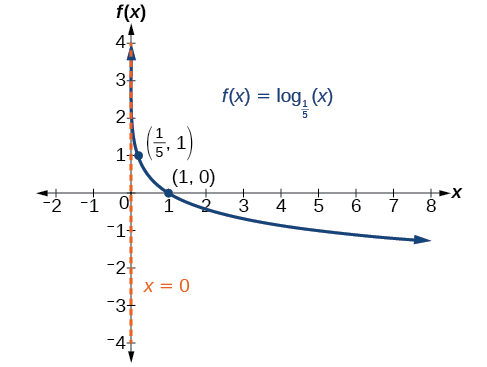# 6.4 Graphs of logarithmic functions  (Page 3/8)

 Page 3 / 8

Given a logarithmic function with the form $\text{\hspace{0.17em}}f\left(x\right)={\mathrm{log}}_{b}\left(x\right),$ graph the function.

1. Draw and label the vertical asymptote, $\text{\hspace{0.17em}}x=0.$
2. Plot the x- intercept, $\text{\hspace{0.17em}}\left(1,0\right).$
3. Plot the key point $\text{\hspace{0.17em}}\left(b,1\right).$
4. Draw a smooth curve through the points.
5. State the domain, $\text{\hspace{0.17em}}\left(0,\infty \right),$ the range, $\text{\hspace{0.17em}}\left(-\infty ,\infty \right),$ and the vertical asymptote, $\text{\hspace{0.17em}}x=0.$

## Graphing a logarithmic function with the form f ( x ) = log b ( x ).

Graph $\text{\hspace{0.17em}}f\left(x\right)={\mathrm{log}}_{5}\left(x\right).\text{\hspace{0.17em}}$ State the domain, range, and asymptote.

Before graphing, identify the behavior and key points for the graph.

• Since $\text{\hspace{0.17em}}b=5\text{\hspace{0.17em}}$ is greater than one, we know the function is increasing. The left tail of the graph will approach the vertical asymptote $\text{\hspace{0.17em}}x=0,$ and the right tail will increase slowly without bound.
• The x -intercept is $\text{\hspace{0.17em}}\left(1,0\right).$
• The key point $\text{\hspace{0.17em}}\left(5,1\right)\text{\hspace{0.17em}}$ is on the graph.
• We draw and label the asymptote, plot and label the points, and draw a smooth curve through the points (see [link] ).

The domain is $\text{\hspace{0.17em}}\left(0,\infty \right),$ the range is $\text{\hspace{0.17em}}\left(-\infty ,\infty \right),$ and the vertical asymptote is $\text{\hspace{0.17em}}x=0.$

Graph $\text{\hspace{0.17em}}f\left(x\right)={\mathrm{log}}_{\frac{1}{5}}\left(x\right).\text{\hspace{0.17em}}$ State the domain, range, and asymptote.The domain is $\text{\hspace{0.17em}}\left(0,\infty \right),$ the range is $\text{\hspace{0.17em}}\left(-\infty ,\infty \right),$ and the vertical asymptote is $\text{\hspace{0.17em}}x=0.$

## Graphing transformations of logarithmic functions

As we mentioned in the beginning of the section, transformations of logarithmic graphs behave similarly to those of other parent functions. We can shift, stretch, compress, and reflect the parent function $\text{\hspace{0.17em}}y={\mathrm{log}}_{b}\left(x\right)\text{\hspace{0.17em}}$ without loss of shape.

## Graphing a horizontal shift of f ( x ) = log b ( x )

When a constant $\text{\hspace{0.17em}}c\text{\hspace{0.17em}}$ is added to the input of the parent function $\text{\hspace{0.17em}}f\left(x\right)=lo{g}_{b}\left(x\right),$ the result is a horizontal shift     $\text{\hspace{0.17em}}c\text{\hspace{0.17em}}$ units in the opposite direction of the sign on $\text{\hspace{0.17em}}c.\text{\hspace{0.17em}}$ To visualize horizontal shifts, we can observe the general graph of the parent function $f\left(x\right)={\mathrm{log}}_{b}\left(x\right)\text{\hspace{0.17em}}$ and for $\text{\hspace{0.17em}}c>0\text{\hspace{0.17em}}$ alongside the shift left, $\text{\hspace{0.17em}}g\left(x\right)={\mathrm{log}}_{b}\left(x+c\right),$ and the shift right, $\text{\hspace{0.17em}}h\left(x\right)={\mathrm{log}}_{b}\left(x-c\right).$ See [link] .

## Horizontal shifts of the parent function y = log b ( x )

For any constant $\text{\hspace{0.17em}}c,$ the function $\text{\hspace{0.17em}}f\left(x\right)={\mathrm{log}}_{b}\left(x+c\right)$

• shifts the parent function $\text{\hspace{0.17em}}y={\mathrm{log}}_{b}\left(x\right)\text{\hspace{0.17em}}$ left $\text{\hspace{0.17em}}c\text{\hspace{0.17em}}$ units if $\text{\hspace{0.17em}}c>0.$
• shifts the parent function $\text{\hspace{0.17em}}y={\mathrm{log}}_{b}\left(x\right)\text{\hspace{0.17em}}$ right $\text{\hspace{0.17em}}c\text{\hspace{0.17em}}$ units if $\text{\hspace{0.17em}}c<0.$
• has the vertical asymptote $\text{\hspace{0.17em}}x=-c.$
• has domain $\text{\hspace{0.17em}}\left(-c,\infty \right).$
• has range $\text{\hspace{0.17em}}\left(-\infty ,\infty \right).$

Given a logarithmic function with the form $\text{\hspace{0.17em}}f\left(x\right)={\mathrm{log}}_{b}\left(x+c\right),$ graph the translation.

1. Identify the horizontal shift:
1. If $\text{\hspace{0.17em}}c>0,$ shift the graph of $\text{\hspace{0.17em}}f\left(x\right)={\mathrm{log}}_{b}\left(x\right)\text{\hspace{0.17em}}$ left $\text{\hspace{0.17em}}c\text{\hspace{0.17em}}$ units.
2. If $\text{\hspace{0.17em}}c<0,$ shift the graph of $\text{\hspace{0.17em}}f\left(x\right)={\mathrm{log}}_{b}\left(x\right)\text{\hspace{0.17em}}$ right $\text{\hspace{0.17em}}c\text{\hspace{0.17em}}$ units.
2. Draw the vertical asymptote $\text{\hspace{0.17em}}x=-c.$
3. Identify three key points from the parent function. Find new coordinates for the shifted functions by subtracting $\text{\hspace{0.17em}}c\text{\hspace{0.17em}}$ from the $\text{\hspace{0.17em}}x\text{\hspace{0.17em}}$ coordinate.
4. Label the three points.
5. The Domain is $\text{\hspace{0.17em}}\left(-c,\infty \right),$ the range is $\text{\hspace{0.17em}}\left(-\infty ,\infty \right),$ and the vertical asymptote is $\text{\hspace{0.17em}}x=-c.$

## Graphing a horizontal shift of the parent function y = log b ( x )

Sketch the horizontal shift $\text{\hspace{0.17em}}f\left(x\right)={\mathrm{log}}_{3}\left(x-2\right)\text{\hspace{0.17em}}$ alongside its parent function. Include the key points and asymptotes on the graph. State the domain, range, and asymptote.

Since the function is $\text{\hspace{0.17em}}f\left(x\right)={\mathrm{log}}_{3}\left(x-2\right),$ we notice $\text{\hspace{0.17em}}x+\left(-2\right)=x–2.$

Thus $\text{\hspace{0.17em}}c=-2,$ so $\text{\hspace{0.17em}}c<0.\text{\hspace{0.17em}}$ This means we will shift the function $\text{\hspace{0.17em}}f\left(x\right)={\mathrm{log}}_{3}\left(x\right)\text{\hspace{0.17em}}$ right 2 units.

The vertical asymptote is $\text{\hspace{0.17em}}x=-\left(-2\right)\text{\hspace{0.17em}}$ or $\text{\hspace{0.17em}}x=2.$

Consider the three key points from the parent function, $\text{\hspace{0.17em}}\left(\frac{1}{3},-1\right),$ $\left(1,0\right),$ and $\text{\hspace{0.17em}}\left(3,1\right).$

The new coordinates are found by adding 2 to the $\text{\hspace{0.17em}}x\text{\hspace{0.17em}}$ coordinates.

Label the points $\text{\hspace{0.17em}}\left(\frac{7}{3},-1\right),$ $\left(3,0\right),$ and $\text{\hspace{0.17em}}\left(5,1\right).$

The domain is $\text{\hspace{0.17em}}\left(2,\infty \right),$ the range is $\text{\hspace{0.17em}}\left(-\infty ,\infty \right),$ and the vertical asymptote is $\text{\hspace{0.17em}}x=2.$

#### Questions & Answers

A laser rangefinder is locked on a comet approaching Earth. The distance g(x), in kilometers, of the comet after x days, for x in the interval 0 to 30 days, is given by g(x)=250,000csc(π30x). Graph g(x) on the interval [0, 35]. Evaluate g(5)  and interpret the information. What is the minimum distance between the comet and Earth? When does this occur? To which constant in the equation does this correspond? Find and discuss the meaning of any vertical asymptotes.
Kaitlyn Reply
The sequence is {1,-1,1-1.....} has
amit Reply
circular region of radious
Kainat Reply
how can we solve this problem
Joel Reply
Sin(A+B) = sinBcosA+cosBsinA
Eseka Reply
Prove it
Eseka
Please prove it
Eseka
hi
Joel
June needs 45 gallons of punch. 2 different coolers. Bigger cooler is 5 times as large as smaller cooler. How many gallons in each cooler?
Arleathia Reply
7.5 and 37.5
Nando
find the sum of 28th term of the AP 3+10+17+---------
Prince Reply
I think you should say "28 terms" instead of "28th term"
Vedant
the 28th term is 175
Nando
192
Kenneth
if sequence sn is a such that sn>0 for all n and lim sn=0than prove that lim (s1 s2............ sn) ke hole power n =n
SANDESH Reply
write down the polynomial function with root 1/3,2,-3 with solution
Gift Reply
if A and B are subspaces of V prove that (A+B)/B=A/(A-B)
Pream Reply
write down the value of each of the following in surd form a)cos(-65°) b)sin(-180°)c)tan(225°)d)tan(135°)
Oroke Reply
Prove that (sinA/1-cosA - 1-cosA/sinA) (cosA/1-sinA - 1-sinA/cosA) = 4
kiruba Reply
what is the answer to dividing negative index
Morosi Reply
In a triangle ABC prove that. (b+c)cosA+(c+a)cosB+(a+b)cisC=a+b+c.
Shivam Reply
give me the waec 2019 questions
Aaron Reply

### Read also:

#### Get the best Algebra and trigonometry course in your pocket!

Source:  OpenStax, Algebra and trigonometry. OpenStax CNX. Nov 14, 2016 Download for free at https://legacy.cnx.org/content/col11758/1.6
Google Play and the Google Play logo are trademarks of Google Inc.

Notification Switch

Would you like to follow the 'Algebra and trigonometry' conversation and receive update notifications?ByByByByByBy